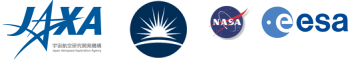Contacts Flare Catalogue Data Search Science Home

## MSSL Data Archive User Guides: EIS Level-2 fits file

EIS Level 2 data provides a quick look first cut at the velocities. Since this is an automated process it is not recommended that you use this for detailed scientific work, but merely as a quick-look to determine if there is anything interested in the data sets such as large velocities. You can obtained the level 2 data and have a look at it online. The level 2 data is produced only for 1" and 2" slit data.

EIS level-2 fits file is generated from level-1 data, so it has same structure (header and data cube) with level-1 data. The user could use conventioanl EIS routine to extract fits header and data cube out from level-2 fits file.

For example:

IDL> eis_getdata, "level-2 filename", wd, (iwin = iwin, /all, hdr = hdr, /ccsds)

The velocity map and width map are stored in the first and second slices of the datcube along Solar-X direction! Sum the left slices together will give out the total intensity map. This is illustrated in the following cartoon figure !

### A short tutorial

#### How to extract Intensity, Velocity and Width map from eis_level2 fits data?

1. First get an eis level-2 fits file from MSSL data archive, for example, eis_l2_20061217_161527.fits.gz (which shows nice loop on the limb).

2. There are two ways to extract the datacube which is contained in this fits file: using conventional "eis_getdata" routines and using "object" routines.

3. "eis_getdata" routine:
• ```IDL> sf='eis_l2_20061217_161527.fits.gz'
IDL> eis_getdata, sf, wd,/all
IDL> help,wd
WD              UINT      = Array[24, 256, 256, 9]
```
here:
• the first dimension of datacube wd ("24") is the spectral window width (ie. pixles on CCD X-direction)
• the second dimension ("256") is the spectral window height (ie. pixels on CCD Y-direction). This is also the value of Solar-Y (arcsec)
• the third dimension ("256") is the number of exposures. This is also the value of Solar-X (arcsec), if it is a raster scanning study in this fits file.
• the fourth dimension ("9") is the number of line winodws included in this fits file. In this case, the line windows are (you need other EIS routines to extract this information):
```	    line_00_FE_X_184.540
line_01_FE_VIII_185.210
line_02_FE_XI_188.230
line_03_CA_XVII_192.820
line_04_FE_XII_195.120
line_05_FE_XIII_202.040
line_06_HE_II_256.320
line_07_FE_XIV_274.200
line_08_FE_XV_284.160
```
• To get velocity, width and intensity maps:

• ```		;this gives you a datacube only for FeXII 195.12
IDL> line04_Fe195=wd[*,*,*,4]
;this gives you velocity map
IDL> line04_Fe195_vel=line04_Fe195[0,*,*]
;this gives you width map
IDL> line04_Fe195_wid=line04_Fe195[1,*,*]
;this gives you intensity map
IDL> line04_Fe195_int=total(line04_Fe195[2:*,*,*],1)
```
• To show these images, using:

• ```               ;load velocity color table
;show velocity map (figure A)
IDL> plot_image,rotate(reform(line04_Fe195_vel),1),\$
/vel,title='velocity'
;show width map (figure B)
IDL> plot_image,rotate(reform(line04_Fe195_wid),1),\$
/vel,title='width'
;show intensity map (figure C)
IDL> plot_image,rotate(reform(line04_Fe195_int),1),\$
title='intensity'
```

• WATCH OUT:

• These figures shown here are a little different from the real velocity. width and intensity maps. This is because that the returned data cube from "eis_getdata" routine is "UINT" datatype, however, the original datacube is "FLOAT" datatype.

4. A better way: using EIS "object" routines

• ```       IDL> sf='eis_l2_20061217_161527.fits.gz'
;create EIS object
IDL> data=obj_new('eis_data',sf)
;see how many lines in
IDL> print,data->getnwin()
9
;get line 4 (FeXII 195.12) datacube
IDL> data->getwin,4,wd
IDL> help,wd
WD	       FLOAT	 = Array[24, 256, 256]
;please notice wd now is FLOAT datatype!
```
• To get velocity, width and intensity maps:

• ```               ;assign wd to line04_Fe195 datacube
IDL> line04_Fe195=wd

;this gives you velocity map
IDL> line04_Fe195_vel=line04_Fe195[0,*,*]
;this gives you width map
IDL> line04_Fe195_wid=line04_Fe195[1,*,*]
;this gives you intensity map
IDL> line04_Fe195_int=total(line04_Fe195[2:*,*,*],1)
```
• To show these images, using:

• ```               ;load velocity color table
;show velocity map (figure D)
IDL> plot_image,rotate(reform(line04_Fe195_vel),1),\$
/vel,title='velocity'
;show width map (figure E)
IDL> plot_image,rotate(reform(line04_Fe195_wid),1),\$
/vel,title='width'
;show intensity map (figure F)
IDL> plot_image,rotate(reform(line04_Fe195_int),1),\$
title='intensity'
```

5. For Intensity map, the current unit is : "erg/cm2/s/sr/Ang", user needs the spectral dispersion to convert the unit to "erg/cm2/s/sr"
```			;dispersion, detector A (long wavelength)
disp.a = 0.022332
;dispersion, detector B (short wavelength)
disp.b = 0.022317
```

6. EIS level-2 data is generated from level-1 data by 'moment' calculation.

However, the datacubes inside level-2 fits file are clean, de-biased, and cosmic-ray-removed data, So user could apply his/her own velocity & width calculation programs, eg. using Gaussin fitting, to suit special demands.

Last Revised: Monday, 24-Jul-2006